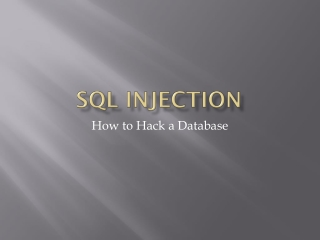# SQL Injection - PowerPoint PPT PresentationDownload PresentationSQL Injection

SQL InjectionDownload Presentation## SQL Injection

- - - - - - - - - - - - - - - - - - - - - - - - - - - E N D - - - - - - - - - - - - - - - - - - - - - - - - - - -
##### Presentation Transcript

1. SQL Injection How to Hack a Database

2. Overview • What is SQL? • Database Basics • SQL Insert Basics • SQL Select Basics • SQL Where Basics • SQL AND & OR Basics • SQL Update Basics • SQL Delete Basics • SQL Injection Basics

3. SQL – What Is It? • Basic Database Functions • Structured Query Language • Common Language For Varity of Databases • ANSI Standard • Database Specific Extensions • Uses Common Baseline Syntax • Scripting Language • Allows Comments (--) • Semicolon Terminates Command (;)

4. SQL – What Is It? • Pros: • Very Flexible • Universal (Oracle, SQL Server, MySQL) • Relatively Few Commands to Learn • Cons: • Requires Detailed Knowledge of the Structure of the Database • Can Provide Misleading Results

5. Database Basics • Four Basic Operations • CRUD • C – Create (Insert) • R – Read (Select) • U – Update • D – Delete

6. SQL Basics – Insert • INSERT – Allows Data to be Inserted into Database • Three Basic Components • Table • Column(s) • Values

7. SQL Basics – Insert • Syntax • INSERT INTO table (column(s)) VALUES (value(s)) • Table – Name of Table Data is Being Stored In • Column(s) – Name of Column, or Columns, to Insert Data Into • Value(s) – Values to Insert • Note: Columns and Values Must be in Same Order

8. SQL Basics - Select • Select – Select Data from Database • Syntax • SELECT column(s) FROM table WHERE condition • Column(s) – Column, or Columns, Names to Retrieve • “*” – Means All Columns from table • Table – Table Name to Get Data From • Can be more than one table

9. SQL Basics - Select • Example • Select state_name, state_abbr FROM states • Select * FROM agencies

10. SQL Basics - Where • Where Clause • Added to Refine Result Set • Uses Conditional Operators • =,>,>=,<,<=,!=(<>) • Between x AND y • IN (list) • LIKE ‘%string’ (“%” us a wild-card) • IS NULL • NOT {BETWEEN / IN / LIKE / NULL}

11. SQL Basics - Where • Examples • SELECT * FROM annual_summaries WHERE sd_duration_code = ‘1’ • SELECT state_name FROM states WHERE state_population > 15000000 • SELECT * FROM annual_summaries WHERE sd_duration_code IN (‘1’,’W’,’X’) AND annual_summary_year = 2000

12. SQL Basics – AND & OR • Multiple WHERE conditions are Linked by AND / OR Statements • “AND” – All Conditions True • “OR” – At Least One Condition is TRUE • Group with ()

13. SQL Basics - Update • Allows Changes to Row(s) of Data in a Table • Three Basic Parts • Name of Table to Update • Column Name to Update • Value to Update • Can Update More Than One Column at a Time • Can Include Where Clause to More Refined Update

14. SQL Basics - Update • Syntax • UPDATE table SET column = value WHERE column = value • Example • UPDATE clubs SET ClubName = ‘Club 1’ WHERE ClubID = 1

15. SQL Basics – Delete • Allows for Data to be Removed from the Database • One Required Part • Table Name • Can Delete All Data in Table, or Just Selected Data • One Optional Part • WHERE Clause – Allows for Selective Delete

16. SQL Basics – Delete • Syntax • DELETE FROM table WHERE column = value • Table – Name of Table to Remove Data from • Column – Name of Column in Table • Value – Value that is in the Column • Example • DELETE FROM clubs (Deletes all Data in Table) • DELETE FROM clubs WHERE ClubID = 1

17. SQL Injection Basics • SQL Takes Advantage of Poor Programming • Inserting SQL Commands into Input Field for Exploitation • Example User Name / Password Input (admin, admin) Into SQL: • SELECT * FROM users WHERE username = ‘admin’ AND password = ‘admin’ • Returns Data for User admin Where Password is admin

18. SQL Injection Basics • SQL Injection Input (admin, ‘ or 1 = 1 --) • SELECT * FROM users WHERE username = ‘admin’ AND password = ‘’ or 1 = 1 -- • Returns Data for User admin Where Password is Empty OR 1 = 1 (Always True) • Note: This will Return All Data in Table

19. SQL Injection Basics • Can Create New User • Using Same User Name / Password Example • Input (admin, ’;INSERT INTO Users VALUES ('Hijack','This') -- • SQL • SELECT * FROM users WHERE username = ‘admin’ AND password = ’’;INSERT INTO Users VALUES ('Hijack','This') -- • Note: Creates a New User (Hijack) with a Password (This)

20. SQL Injection Basics • Can Create Table Values • Using Same User Name / Password Example • Input (admin, ’;UPDATE Orders Set Amount=0.01-- • SQL • SELECT * FROM users WHERE username = ‘admin’ AND password = ’’;UPDATE Orders Set Amount=0.01-- • Note: Sets all Order Amounts to one cent

21. References • SQL • http://w3schools.com/sql/sql_syntax.asp • http://www.teach-ict.com/as_as_computing/ocr/H447/F453/3_3_9/sqlintro/miniweb/index.htm • SQL Injection • http://zerofreak.blogspot.com/2012/01/chapter2-basic-sql-injection-with-login.html • Practice Site • http://google-gruyere.appspot.com/